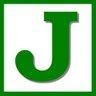# Electric ForcePaul Jennings
Get Started. It's FreeElectric Force## 1. model of the atom

### 1.1. electron cloud model

1.1.1. nucleus

1.1.1.1. center composed of neutrons and protons

1.1.2. electron cloud

1.1.2.1. where electrons will be found 90% of the time

2.1.1. protons

2.1.1.1. p+

2.2.1. electrons

2.2.1.1. e-

2.3.1. neutron

## 3. charge can transfer through materials

### 3.1. conductors

3.1.1. easily transfer electric charges

### 3.2. insulator

3.2.1. electric charges transfer with great difficulty

3.2.1.1. electrostatic discharge occurs when a gas is temporarily turned into a plasma due to a large electric force.

3.2.1.1.1. examples

## 4. Coulomb's Law

### 4.3. Fc = kc q1 q2 / r^2

4.3.1. kc = 8.99 x 10^9 Nm^2/C2

4.3.2. q - net electric charge

4.3.3. r - distance between charges

4.3.4. Fc - Electric Force

## 5. objects are normally electrically neutral

### 5.1. conduction

5.1.1. charges physically are transferred between objects

### 5.2. induction

5.2.1. charge in an object becomes polarized due to the presence of a large electric force